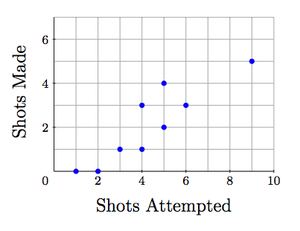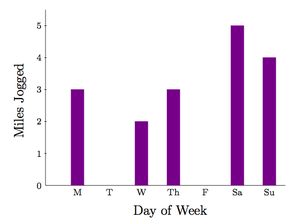# Introducing Functions

Alignments to Content Standards: 8.F.A 8.F.A.1

1. For each situation below, fill in the missing information in the tables.
1. A parking meter takes only dimes and each dime is worth 6 minutes on the meter.

Number of Dimes Minutes of Parking
2
3
7
Minutes of Parking Number of Dimes
0
12
54
2. Each point on the graph below shows how many shots a player on a basketball team took and made in the first half of a game.2
4
7
1
4
6
3. Below is a graph showing how far Marika jogged each day in a week:Day of Week Miles Jogged
Monday
Friday
Sunday
Miles Jogged Day of Week
1
3
5
2. What, if any, difficulties did you encounter filling out these tables? Explain.

## IM Commentary

The goal of this task is to motivate the definition of a function by carefully analyzing some different relationships. In some of these relationships, one quantity can be determined in terms of the other while in others this is not possible. In this way, students are led to see what is special about a function, namely that to each input there corresponds one and only one output. The statement of the task is ambiguous for examples (ii) and (iii). There are at least two valid approaches open to students:

• Not entering any value in the table if there is not a single, well determined value,
• Entering all appropriate values.

In some ways, the latter approach is more informative because then the table is seen as a different way of presenting the relationship under examination. The variable in the second column of a table is called a function of the variable in the first column when there is exactly one entry in the second column for each entry in the first column.

Below is an example the teacher may wish to use where it is not feasible to give a complete list in one of the tables:

City State
Baton Rouge
Seattle
Honolulu

State City
Massachusetts
Iowa
Texas

The first table can be filled out by listing the states in which these three cities are found (Louisiana, Washington, Hawaii). For different city names, such as Springfield or Jackson, there would be many states having a city with that name. The second table cannot be filled out readily as we would need to know every city in these states.

The teacher may wish to point out that scenario (i) is very different from (ii) and (iii) in the sense that there is a mathematical rule which relates the number of dimes to the number of minutes in an unambiguous way: for $x$ dimes, we get $6x$ minutes of parking and for $y$ minutes of parking (where $y$ is a multiple of 6) we needed to insert $\frac{y}{6}$ dimes.

## Solution

1.
1. Since each dime gives 6 minutes on the parking meter, we can multiply the number of dimes by 6 to find how many minutes of parking we will get:

Number of Dimes Minutes of Parking
2 12
3 18
7 42

Since each dime gives 6 minutes of parking, the number of dimes inserted will be $\frac{1}{6}$ the number of minutes on the parking meter:

Minutes of Parking Number of Dimes
0 0
12 2
54 9
2. For the first row of the table, only one player attempted 2 shots, making 0 of them, so we enter a zero in the second column. Two players attempted 4 shots and they made 1 shot and 3 shots respectively. No player attempted 7 shots and so this box must be left blank.

2 0
4 1,3
7

The entries in the table where there were two possiblities or no possibilities have been highlighted. The entry with two possibilities could be left blank if we take the point of view that we should either fill in a box in the table with a single value or not fill it in at all.

Following the same method, we can fill out the next table:

1 3, 4
4 5
6
3. For the first table, we read from the graph how many miles Marika jogged each day:

Day of Week Miles Jogged
Monday 3
Friday 0
Sunday 4

For the second table, we run into some problems like on part (ii) and have filled out the table using the same method:

Miles Jogged Day of Week
1
3 M, Th
5 S
2. There is no problem filling out any of the tables if we allow no entries or multiple entries. If, however, we want a single entry in the right hand column of the table (just like there is always a single entry in the left hand column) then there are multiple problems. There are some rows where there is nothing to enter in the right had column: for example, Marika never ran 1 mile on any day and no player made 6 shots. There are also some rows with multiple entries in the second column: Marika ran 3 miles on both Monday and Thursday and two players made a single shot in the basketball game.

Carrying this analysis a little further, in the first example, for any number of dimes placed in the meter, the minutes of parking will be 6 times this amount. For any number of minutes of parking (which is a multiple of 6) the number of dimes put in the meter will be $\frac{1}{6}$ this amount. For the basketball example, some numbers of shots attempted (1, 2, 3, 6, 9) tell us how many shots were made while others (4, 5) do not. Some numbers of shots made (2, 4, 7) tell us how many shots were attempted while others (0, 1, 3) do not. In the third scenario, for each day of the week we know how far Marika jogged. There were multiple days when she jogged zero and 3 miles but only one day of the week when she ran 2, 4, and 5 miles.